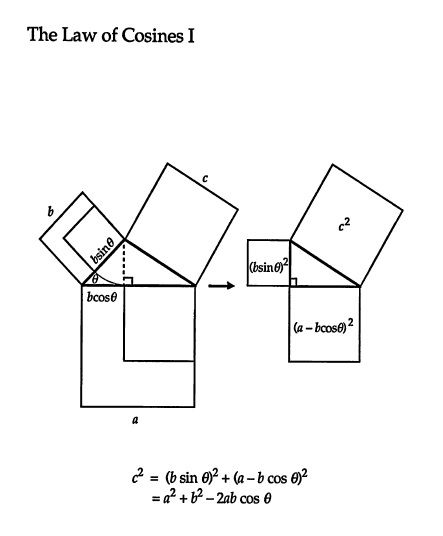TrigonometryCompletion of the proof of the Cosine Rule

Prove It

Well-known member
MHB Math Helper
Hello my friends,

I posted this picture as a proof of the Cosine Rule in another thread,however after having a closer look at it, I believe it is incomplete. It works by drawing a segment from one of the vertices so that this segment is perpendicular to one side of the triangle, and then applying Pythagoras' Theorem.

However, if you have an obtuse-angled triangle, it is impossible to draw a segment from one of the acute vertices to make a right-angle triangle. So how is it possible to prove this relationship:

$$\displaystyle \displaystyle c^2 = a^2 + b^2 - 2\,a\,b\cos{(C)}$$

when C is an obtuse angle?